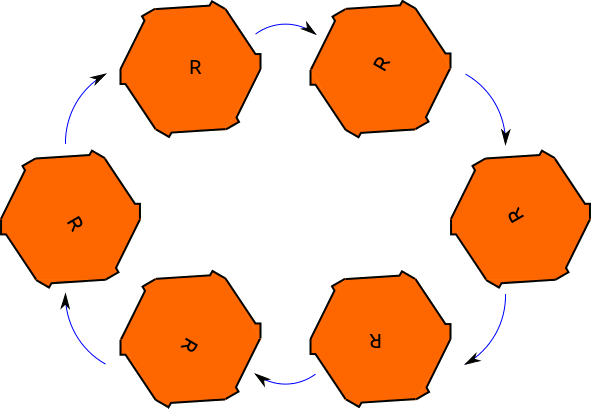Skip to main content
$$\newcommand{\id}{\mathrm{id}}$$ $$\newcommand{\Span}{\mathrm{span}}$$ $$\newcommand{\kernel}{\mathrm{null}\,}$$ $$\newcommand{\range}{\mathrm{range}\,}$$ $$\newcommand{\RealPart}{\mathrm{Re}}$$ $$\newcommand{\ImaginaryPart}{\mathrm{Im}}$$ $$\newcommand{\Argument}{\mathrm{Arg}}$$ $$\newcommand{\norm}{\| #1 \|}$$ $$\newcommand{\inner}{\langle #1, #2 \rangle}$$ $$\newcommand{\Span}{\mathrm{span}}$$

# 1.3: Abstraction. What is a group?

$$\newcommand{\vecs}{\overset { \rightharpoonup} {\mathbf{#1}} }$$

$$\newcommand{\vecd}{\overset{-\!-\!\rightharpoonup}{\vphantom{a}\smash {#1}}}$$

So we now have many examples of symmetry, but what, exactly is a group?

First, let's remember where numbers come from. You can imagine having a set of three pineapples, or a set of three people, or a set of three bottles. All of these share the property of 'three-ness.' So when we say the number three, it describes all of these different sets. The number three is an abstraction of the property of a set containing three things.

What's a group? A group is an abstraction of symmetry! Just like a number describes all sets with a certain number of things in it, a given group describes all objects with a certain kind of symmetry. Here's an example: The 'very symmetrical face' has two symmetries, related by a reflection. Likewise, the symmetric polynomial $$f(x,y)=x+y$$ has a two symmetries, one from leaving $$f$$ alone, and one from exchanging the two variables $$x$$ and $$y$$. If we think of switching $$x$$ and $$y$$ as a reflection, we see that the face and the polynomial somehow have the same kind of symmetry! The group is that measure of symmetry.

Now, in that last example, the two objects had the same number of symmetries. It turns out that just counting symmetries ins't enough to tell whether the two objects have the same group of symmetries. Think of the symmetries of our equilateral triangle: there were rotational symmetries, and there was a reflection symmetry. And there were six symmetries in all. Now, consider a regular hexagon with some regularly placed bumps on each side, so that there is no reflection symmetry available. Thus, this object has six rotational symmetries, but can't be flipped over like the triangle. Therefore it has the same number of symmetries as the equilateral triangle, but the set of symmetries is somehow different.The six rotational symmetries of the bumpy hexagon. The blue arrow represents the rotation $$r$$; $$r^6=id$$.

## Contributors

• Tom Denton (Fields Institute/York University in Toronto)